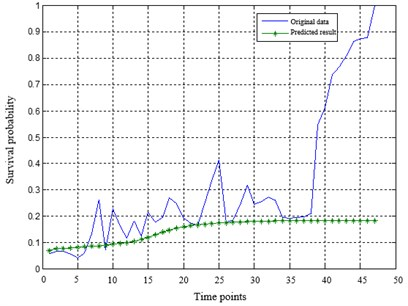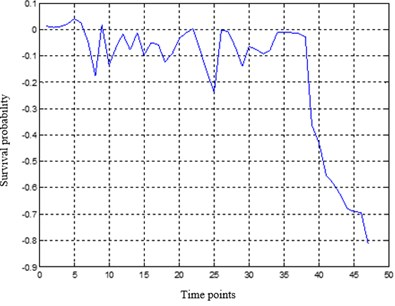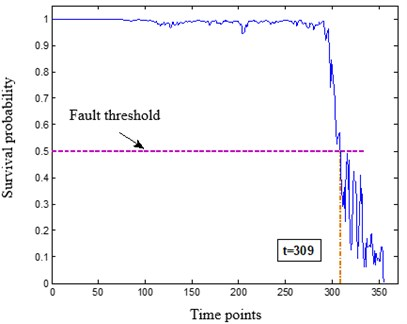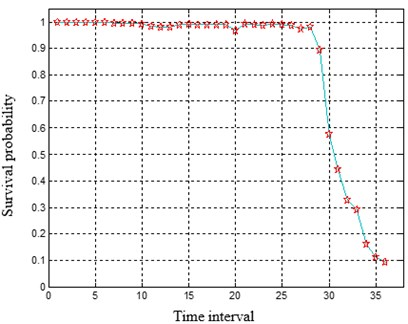Published: 02 December 2021

# A remaining useful life prediction method for engineering components based on intelligent product limit estimator

Xiaosheng Zeng1
Pian Hu2
Xiang Yu3
Taotao Zhou4
Jiangyuan Liu5
1, 3College of Naval Architecture and Ocean Engineering, Naval University of Engineering, Wuhan, China
2, 4, 5China Ship Development and Design Center, Wuhan, China
Corresponding Author:
Taotao Zhou
Views 37

#### Abstract

Aiming at predicting the remaining useful life of key components of engineering equipment, a remaining useful life prediction method based on an intelligent product limit estimator is developed. The proposed approach can overcome the shortcoming that current life prediction methods require a large number of life data values, as well as condition monitoring data and life cycle data. Besides, to solve the problem of “completely truncated data” in the prediction of key components, the life estimation value of the predicted object is obtained by using the fitting residuals, and the survival probability of the predicted object in a period in the future is obtained. A case study on the spindle bearing of a certain type of water pump shows the effectiveness of the proposed approach.

## 1. Introduction

Once the key components of typical products fail or degenerate, the system function may be reduced or lost, or even lead to catastrophic accidents. Therefore, it is necessary to predict the residual life of key components of typical products. The major task of Remaining useful life (RUL) prediction is to forecast the time left before the machinery losses its operation ability based on the condition monitoring information. Scholars have done a lot of research on RUL prediction. Giorgio et al.  combined the Markov process and a gamma process to establish a cylinder liner wear degradation model; Liu et al.  proposed a non-homogeneous continuous-time Markov model to predict the RUL of multi-state systems, and optimized the replacement strategy according to the prediction results; Sbarufatti et al.  combined Feed-forward neural networks (FFNNs) with sequential Monte-Carlo sampling to predict RUL of a fatigue crack; Pan et al.  and Xiao et al.  used FFNN to predict the bearing health state in advance; Zemouri et al.  proposed a recursive radial basis function network and used it to predict the RUL of machinery; Liu et al.  proposed a method to enhance RNN for RUL prediction by improving the memory property of RNN; Widodo et al.  trained a SVM model using censored data and complete data, and predicted the survival probability of the machine. Benkedjouh et al.  used support vector regression (SVR) to map health indicators to nonlinear regression, and then fitted the regression to power model for mechanical RUL prediction; Liu et al.  developed an improved probabilistic support vector machine (SVM) to predict the degradation process of nuclear power plant components; Fumeo et al.  developed an online support vector machine model for bearing RUL prediction by balancing the accuracy and computational efficiency; Wang et al.  used similarity-based method combined with relevance vector machine (RVM) sparse learning to predict the RUL of the machine. Generally, the above-mentioned work requires a large number of life cycle data to guarantee prediction accuracy.

To improve the accuracy under limited samples, as well as solve the problem of “completely truncated data”, a remaining useful life prediction method based on an intelligent product limit estimator is developed in this work, which aims to improve the accuracy of the prediction of the remaining useful life of the engineering component and provide support for the condition-based maintenance and self-guarantee of typical equipment. A spindle bearing of a certain type of water pump has demonstrated the effectiveness of the proposed approach.

## 2. The proposed method

### 2.1. Kaplan-Meier nonparametric estimation

Kaplan-Meier nonparametric estimation is one type of product limit estimation. This method should be used to find out that both the life data and the right deleted data are known exactly, that is, it cannot be interval data. By observing the lifespan of n individuals of population $T$, we can get ${t}_{1},{t}_{2},\dots {t}_{n}$ (there may be right-censored data, but no left-censored data). When ${t}_{i}$ is terminal data, let ${\delta }_{i}=1$; When ${t}_{i}$ is right-censored data, let ${\delta }_{i}=0$, our data can be recorded as $\left({t}_{i},{\delta }_{i}\right)$, $i=1,2,\dots ,n$.

Rearrange these ${t}_{i}$ from small to large, when a deleted data is equal to a final data, then the end of life data is placed before the deleted data ${t}_{\left(1\right)}\le {t}_{\left(2\right)}\le ...\le {t}_{\left(n\right)}$.

When ${t}_{\left(i\right)}$ is the end of life data, mark ${\delta }_{\left(i\right)}=1$; When ${t}_{\left(i\right)}$ is right-censored data, mark ${\delta }_{\left(i\right)}=0$$\left(1\le i\le n\right)$.

The product limit of $S\left(t\right)$ is defined as follows:

1
$\stackrel{^}{S}\left(t\right)=\left\{\begin{array}{l}1,\mathrm{}\mathrm{}\mathrm{}\mathrm{}\mathrm{}t\in \left[0,{t}_{\left(1\right)}\right),\\ \prod _{i=1}^{j}\left(\frac{n-i}{n-i+1}{\right)}^{{\delta }_{\left(i\right)}},\mathrm{}\mathrm{}\mathrm{}\mathrm{}\mathrm{}t\in \left[{t}_{\left(j\right)},{t}_{\left(j+1\right)}\right),\mathrm{}\mathrm{}\mathrm{}\mathrm{}\mathrm{}\mathrm{}j=1,2,...,n-1,\\ 0,\mathrm{}\mathrm{}\mathrm{}\mathrm{}\mathrm{}t\in \left[{t}_{\left(n\right)},\infty \right).\end{array}\right\$

When $t<{t}_{n}$, $\stackrel{^}{S}\left(t\right)$ has the following unified expression:

2
$\stackrel{^}{S}\left(t\right)=\prod _{{t}_{i}\le t}^{}{\left(\frac{n-i}{n-i+1}\right)}^{{\delta }_{\left(i\right)}}.$

Here, it is agreed that $\prod _{\varnothing }{a}_{i}=1$ and $\varnothing$ is an empty set.

### 2.2. Time series prediction based on parallel multilayer perceptron and residual fitting

Because of the irregularity of chaotic time series, the key part of neural network prediction is to learn the law of data, and then get the network that can best express the law characteristics of data series. The parallel multi-layer perceptron (PMLP) network composed of multiple parallel MLPs is used to predict chaotic time series, which can improve the prediction accuracy and network performance. Here, each MLP has a different number of input nodes, and then the combination weight coefficient of the parallel MLP network is determined by the least square method, for the sake of obtaining the best combination of the number of input nodes.

## 3. Test example

### 3.1. Data acquisition

The performance of the proposed method is verified through the remaining useful life prediction of spindle bearing from the water pump. The bearing type is deep groove ball bearing SKF 6209. The specific parameters of the bearing are: inside diameter 45 mm, outer diameter 85 mm, and thickness 19 mm. The data of the rolling bearing were acquired from a simulation test bed. The horizontal vibration signals of the bearing were collected by the horizontal acceleration sensor with the 2 min recording interval and 25.6 kHz sampling frequency. 40 sets of vibration data were collected, among which 30 sets of truncated data are used as training samples while the remaining 10 full life data samples are used as test samples.

### 3.2. Test results

The verification of the proposed method including three steps: constructing the PMLP network, construction of the survival state parameter table collection, and prediction of residual service life of key components. The detailed steps are illustrated as follows.

Step 1: Performance degradation index sequence prediction based on PMLP network prediction and residual fitting.

Training the PMLP network with the training samples until the specified accuracy is reached. The PMLP network is composed of three subnetworks. The number of hidden layer nodes in MLP network is determined by the empirical formula $a=\sqrt{{n}_{1}+{n}_{2}}+k\left(0\le k\le 10\right)$. Tansig function and purelin function are utilized in the hidden layer and the output layer respectively. The maximum training time is set to 1500. The gradient descent method with momentum and adaptive learning rate is adopted for network training. The optimal weight allocation of the three subnetworks is $\omega =\left[0.5980\mathrm{}\mathrm{}0.402\right]$. Then, the data samples are predicted to obtain the degradation index sequence, whose definition is: residual = the true value of the index – the predicted value of the network. The prediction results of PMLP network is adjusted according to the predicted residual, which is fitted with 4th order polynomial. Fig. 1 shows the predicted results and residual results of the index sequence.

Fig. 1The predicted results and residual results of PMLP networka) The predicted results by the PMLP networkb) The residual results by the PMLP network

Based on the residual error fitting function, the function values of the variables can be obtained and the residuals sequence is extended. Then, the obtained iterative predicted value of degradation index is corrected with the formula “The final predicted value = PMLP network prediction - fitting residual error”.

According to the fitting function of the residual, the residual value of the subsequent points can be obtained by inputting several data values before the truncation point into the trained PMLP neural network for iterative prediction. And then the QE predicted value after the truncation point can be obtained. Finally, the extrapolated residual value was added to the predicted value of QE to correct the predicted value of QE.

The sample data after the truncated points were added and improved, so as to directly obtain the bearing performance degradation characterization parameter set within the whole life cycle. When the health indicator (CV value) is less than a certain threshold, the device is in critical condition. Therefore, when the index is higher than the threshold value of 0.5, the equipment is deemed to have failed. For the truncated data samples after completion, the data points exceeding the settled failure threshold need to be discarded, that is, the truncated data samples after preprocessing need to be fine-tuned.

Step 2: Construction of the survival state parameter table collection based on the product limit estimation.

20 out of the 30 training samples are pretreated by using the above method, namely the degradation index samples of truncated data are supplemented into complete data samples. While the remaining 10 groups of bearing data samples are still truncated data without preprocessing. Based on the intelligent product limit estimation method, the survival state parameter list collections were constructed for the two types of data as follows.

1) The construction method of preprocessed samples.

Find the failed time interval of the samples. For the training samples earlier than the failed time interval, the survival probabilities of them are set to 1. The survival probabilities of the remaining samples are set to 0.

2) The construction method of truncated samples.

The number of failed bearing samples and the number of withdrawn samples in each time interval was counted among the 30 sample sets. The survival probabilities of 10 truncated samples in each time interval are calculated with the K-M estimation rule.

Step 3: Prediction of residual service life of key components based on feedforward neural network.

The residual service life of the bear is predicted based on the feedforward neural network that consists of three layers. The input layer of the network contains seven nodes. The input vectors are respectively the degradation index QE value of T bearing at the current moment and the degradation index value of the previous six moments. The outputs are the survival probability values in the 5 time periods after the current time period. The FFNN network is trained with the back propagation (BP) algorithm. The survival probability curve of any test sample can be drawn in units of time point and time period respectively after predicting with the constructed neural network, as shown in Fig. 2.

Fig. 2The survival probability prediction of a test sample in units of time point and time intervalThe survival probabilities of the 10 bearing test samples are predicted with the intelligent product estimator and listed in Table 1. It can be seen that the relative error between the predicted value and the true value is less than 10 %. To achieve accurate residual life prediction, the halfway point of the bearing in a time interval is regarded as the end points in whole life cycle in this example. Namely when the survival probability prediction result is the $k$-th time interval, the failure time of the test example is $\left(k×10+k×9\right)/2=9.5k$. The confidence interval for failure time prediction is [9k, 10k], and the confidence is larger than 90 %. In summary, the prediction accuracy of typical bearing residual service life based on the intelligent product limit estimator method is higher than 85 % and the confidence is higher than 90 %.

Table 1The prediction results of the survival probability for the 10 test samples

 Test sample No. 31 32 33 34 35 36 37 38 39 40 The real failure time 26 14 31 32 10 20 39 15 25 34 Predict the failure time 27 15 31 31 11 21 38 16 26 34 Accuracy (%) 96.15 92.86 100 96.88 90 95 97.44 93.33 96 100

## 4. Conclusions

To meet the requirements of pre-maintenance and self-support of key components of engineering equipment, a residual service life prediction method based on intelligent product limit estimator is proposed in this paper. While establishing the performance degradation index of key components, the life estimation value of the key components is obtained by using the fitting residual. The survival probability of the predicted object in a period of time in the future is obtained with completely truncated condition monitoring data and failure data. The proposed method can overcome the shortcomings that the current life prediction methods need a large number of life data values, condition monitoring data and life cycle data. Through case verification, the prediction accuracy of typical bearing residual service life based on intelligent product limit estimator method is higher than 85 %, and the confidence is higher than 90 %.